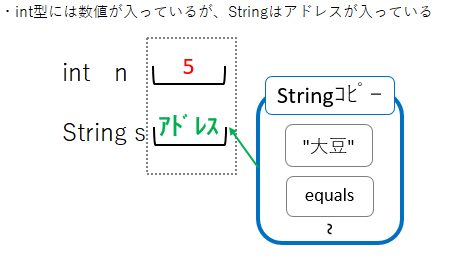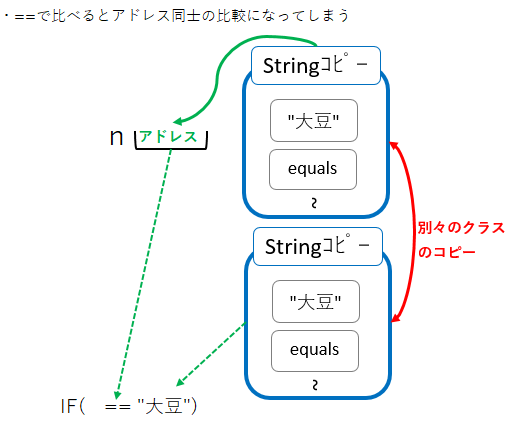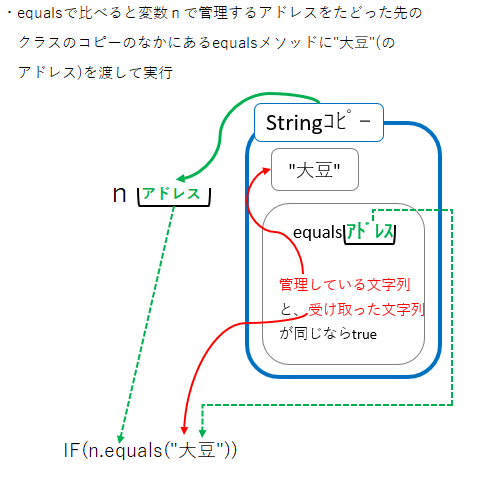# String class comparison

There are occasions when you use a comparison of equal values in if statements, etc. For example ...

【Q1】 A program that checks if the number you enter matches the answer

#### `Q1.java`

``````
import java.util.Scanner;

class Q1 {
public static void main(String[] args) {
Scanner stdIn = new Scanner(System.in);

System.out.print("Enter the number:");
int n = stdIn.nextInt();

if (n == 5) {
System.out.print("Per");
} else {
System.out.print("Off");
}
}
}

``````

In this case, it is a comparison of int type numbers. You can compare using ==. Now, let's look at the following case as a comparison of strings.

【Q2】 Mystery program

#### `Q1.java`

``````
import java.util.Scanner;

class Q2 {
public static void main(String[] args) {
Scanner stdIn = new Scanner(System.in);

System.out.print("What are the ingredients for tofu? :");
String n = stdIn.next();

if (judge.equals("soy")) {
System.out.print("Per");
} else {
System.out.print("Off");
}
}
}

``````

I'm using equals for comparison instead of ==. The reason for equals is ...

If you use == in a class type (reference type) comparison, it's not the content It will be a comparison of the "address" that contains the data.